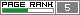Sunday, June 05, 2005

The DeJong (De Jong) Test Suit F2

Hallo!! :D

Here another function from the The DeJong Test Suit.

The function is this:

F(x1,x2) = 100*(x1^2 - x2)^2 + (1 - x1)^2

The function is very simple and I got good values. The problem is to minimize the function. The global minimum is f(x1,x2) = 0 at (x1,x2) = (1,1).

ES Configuration:

Population = 60
Offspring = 60 (I used the (mu+mu)ES )
Generation = 5000
Simulation Time = 9.493 s

Below we have the function's graph:Another view:Now you see the ES performance. The horizontal axis is the number of generations ant the vertical axis is the best fitness.A closer image from the image above:The initial at first generation's values that I got were:

F(x1,x2) = 1.2143242479659295
x1 = -0.02275919812830051
x2 = 0.041540885751586681

The final values were:

F(x1,x2) = 1.6182695892245374e-008
x1 = 1.000118812318453
x2 = 1.0002330930642427

A good aproximation. :D

See You!!

Nosophorus

P.S: Next post I will show the Colville Function. This one has four variables. :D